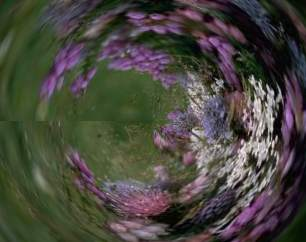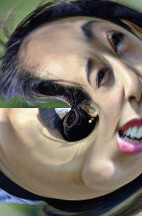## TUNNELIZE

 Creates a tunnel-like effect on an image.

 USAGE: tunnelize [-m mode] infile outfile USAGE: tunnelize [-h or -help] -m .... mode ..... mode of transform; mode=1 or 2; default=1 PURPOSE: To create a tunnel-like effect on an image. DESCRIPTION: TUNNELIZE creates a tunnel-like effect on an image by swapping polar for cartesian coordinates. ARGUMENTS: -m mode ... MODE is either 1 or 2. Mode=1 swaps angle for x and radius for y. Mode=2 swaps radius for x and angle for y. NOTE: This script may run rather slowly as it uses -fx. A similar effect to mode=1 can be achieved using -distort arc "360 90 size 0", where size is in the range of half one or the other dimensions of the image or as large as the half diagonal of the image. CAVEAT: No guarantee that this script will work on all platforms, nor that trapping of inconsistent parameters is complete and foolproof. Use At Your Own Risk.

### EXAMPLES

 Image Tunnelization http://www.cescg.org/CESCG97/boros/ OriginalArguments: -m 1 Arguments: -m 2Comparison To -Distort Arc Arguments: -distort arc "360 90 121 0" (height/2 = 121) Arguments: -distort arc "360 90 160 0" (width/2 = 160)Image Tunnelization OriginalArguments: -m 1 Arguments: -m 2What the script does is as follows: Calculates the polar coordinates from the cartesian coordinates Scales the cartesian coordinates by the ratio of the polar coordinates divided by the maximum polar coordinates This is equivalent to the following IM commands for the case of -m 1. w2=`convert \$infile -format "%[fx:w/2]" info:` h2=`convert \$infile -format "%[fx:h/2]" info:` r2=`convert \$infile -format "%[fx:hypot(w/2,h/2)]" info:` pi2=`convert xc: -format "%[fx:2*pi]" info:` convert \$infile -monitor \ -fx "xx=(i-\$w2); yy=(j-\$h2); rr=hypot(xx,yy); aa=atan2(yy,xx); u.p{(aa+pi)*w/\$pi2,rr*h/\$r2}" \ \$outfile